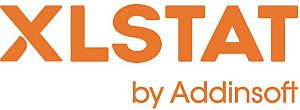# XLSTAT - Multivariate Analysis of Variance (MANOVA)

## What is MANOVA?

Multivariate ANalysis of VAriance (MANOVA) uses the same conceptual framework as ANOVA. It is an extension of the ANOVA that allows taking a combination of dependent variables into account instead of a single one. With MANOVA, explanatory variables are often called factors.

The advantage of the MANOVA as opposed to several simultaneous ANOVAs lies in the fact that it takes into account correlations between response variables which results in a richer use of the information contained in the data. The combination of dependent variables may represent a variable that cannot be measured directly.

The MANOVA tests the effect of factors on several response variables. MANOVA also enables the simultaneous tests of all hypotheses tested by an ANOVA and is more likely to detect significant factor effects.

Furthermore, the computation of several ANOVAs instead of one MANOVA increases the Type I error which is the probability to wrongly reject the null hypothesis.

The potential covariation between response variables is not taken into account by several ANOVAs. Instead, the MANOVA is sensitive to both the difference of averages between levels of factors and the covariation between explanatory variables. And a potential correlation between response variables is more likely to be detected when these variables are studied together as it is the case with a MANOVA.

Let’s consider as an illustrative example a two-way MANOVA. The model is written as followed:

yijk = μ + αi + βj + αβij + εijk (1)

Where yijk is the kth observation of the ith level of A and jth level of B, e is the error of the model.

The hypotheses used in a MANOVA are identical to those used in linear regression: errors eifollow a normal distribution N(0,s) and are independent.

To use the various tests proposed in the results, it is recommended to check retrospectively that the underlying hypotheses have been correctly verified. The normality of the residuals can be checked by analyzing certain charts or by using a normality test. The independence of the residuals can be checked by analyzing certain charts or by using the Durbin-Watson test.

## MANOVA Options in XLSTAT

One of the main application of the MANOVA is multivariate comparison testing where parameters for the various categories of a factor are tested to be significantly different or not. For example, in the case where four treatments (factors) are applied to plant fields, we want to know if the factors and/ or their interactions have a significant effect on a combination of dependent variables depicting production quality.

Numerous tests have been proposed for this purpose. Most of them rely on the relationships that exist between the error matrix E and the matrix symbolizing the tested hypotheses H that is the eigenvalues of the matrix E-1H. XLSTAT provides the following tests:

Wilks Lambda test

Hotelling-Lawley’s Trace test

Pillai’s Trace test

Roy’s greatest root test

## MANOVA Results in XLSTAT

Summary statistics: The tables of descriptive statistics show the simple statistics for all the variables selected. The number of observations, missing values, the number of non-missing values, the mean and the standard deviation (unbiased) are displayed for the dependent variables (in blue) and the quantitative explanatory variables. For qualitative explanatory variables the names of the various categories are displayed together with their respective frequencies.

SSCP matrices: These tables are displayed to give a general view of the effects of the factors and interactions between factors.

When a k-way MANOVA is applied and the corresponding option is enabled, the results of the multivariate tests are displayed. The associated statistics, the degrees of freedom and the p-values are displayed.This analysis is available in the XLStat-Basic addin for Microsoft ExcelKovach Computing Services (KCS) was founded in 1993 by Dr. Warren Kovach. The company specializes in the development and marketing of inexpensive and easy-to-use statistical software for scientists, as well as in data analysis consulting.

### Get in Touch

• Email:
sales@kovcomp.com